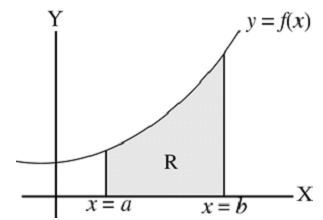# Definite Integrals

The beauty and importance of the integral calculus is that it provides a systematic way for the exact calculations of many areas, volumes and other quantities.

Consider the region R in the plane. The region R is bounded by the curve y= f(x), x-axis and two vertical lines x = a and x = b, where b > a.

Assume that y = f(x) is a continuous and increasing function on the closed interval [a, b].Let ∫ y dx = F(x) + c

If x = a, then the area up to x= a, Aa is

∫ y dx = F(a) + c

If x = b, then the area up to x= b, Ab is

∫ y dx= F(b) + c

The required area of the region R is

Ab − Aa = = (F(b) + c) − (F(a) + c)

ab y dx = F(b) − F(a)

ab f(x) dx = F(b) − F(a)

This integration gives the area of the region R bounded by the curve y = f(x), x axis and between the lines x = a and x = b. a & b are called the lower and upper limits of the integral.